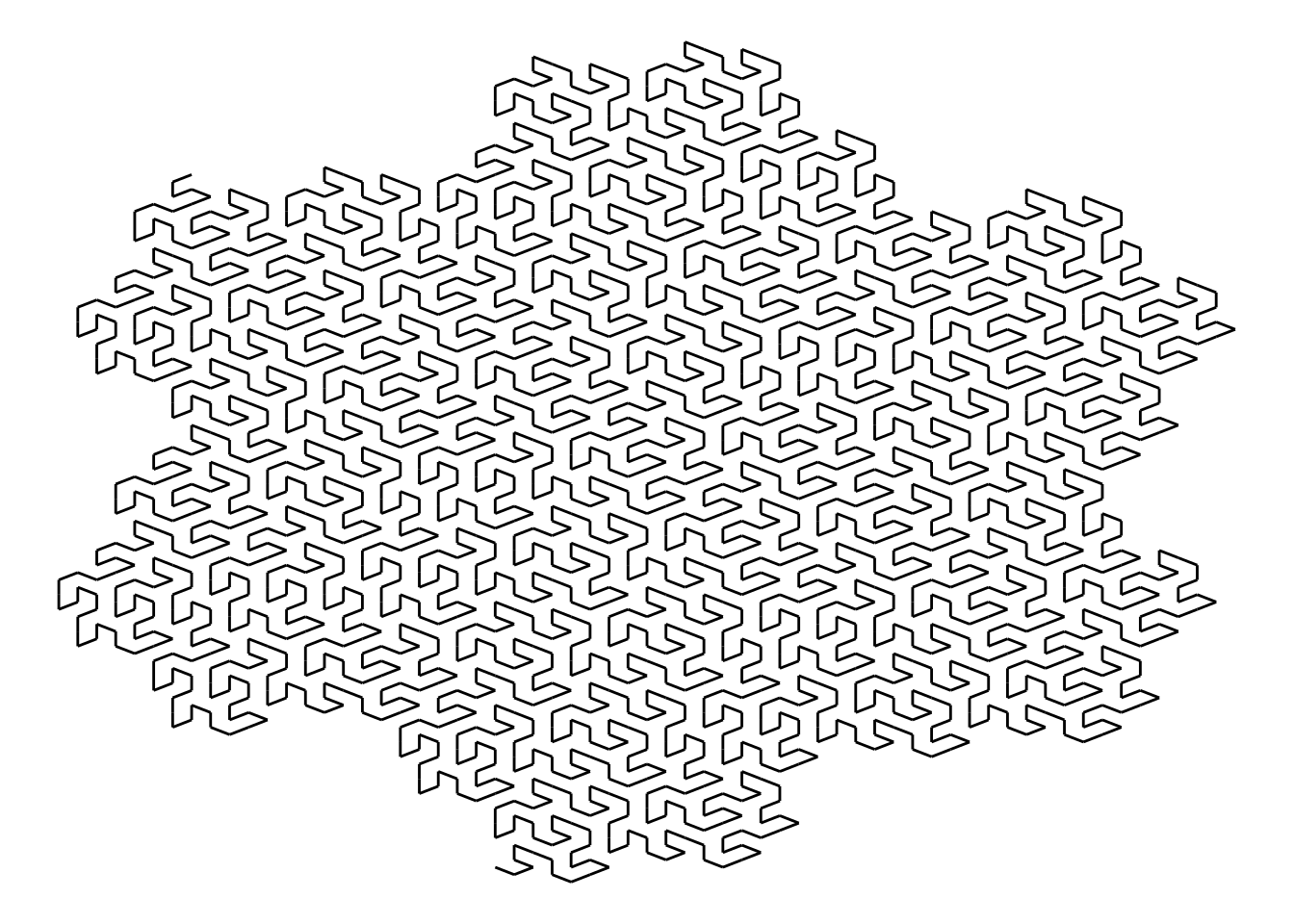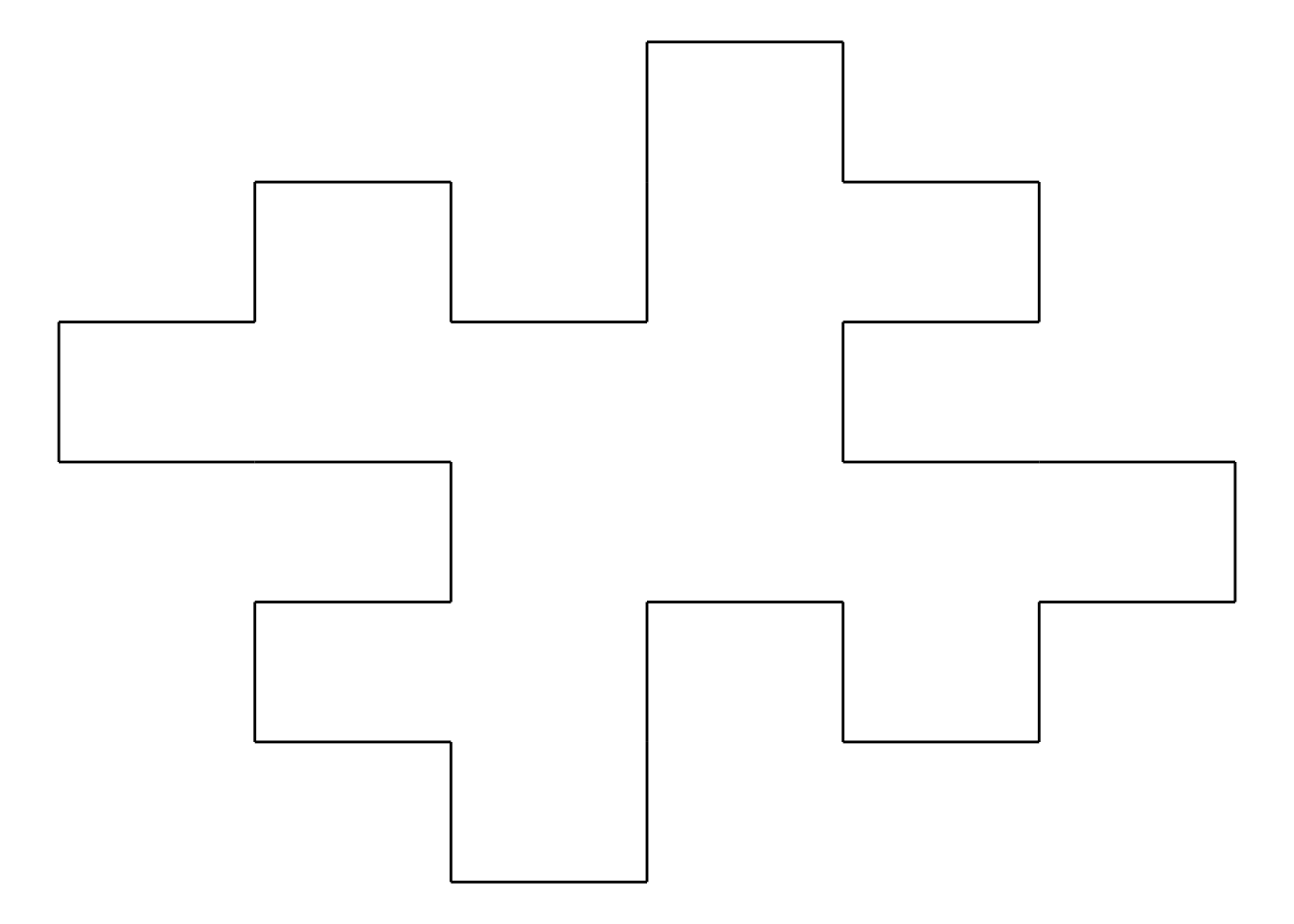## Automatically cropping images

As mentioned in previous posts, I need numerous images to train an image recognition model. My goal is to have many examples of the image of Elizabeth II like the one below. To be efficient, I want to process many photographs of 1 cent coins using a program and so that program must be able to reliably find the centre of the portrait.

To crop the image I used two methods: 1. remove whitespace from the outside inward and 2. find the edge of the coin using OpenCV’s cv2.HoughCircles Function.

Removing whitespace from the outside inward is the simpler of the two methods. To do this I assume the edges of the image are white, color 255 in a grayscale image. If the mean value of pixel colors is 255 for a whole column of pixels, that whole column can be considered whitespace. If the mean value of pixel colors is lower than 255 I assume the column contains part of the darker coin. Cropping the image from the x value of this column will crop the whitespace from the left edge of the image.

``````for img_col_left in range(1,round(gray_img.shape/2)):
if np.mean(gray_img,axis = 0)[img_col_left] < 254:
break ``````

The for loop of this code starts from the first pixel and moves toward the center. If the mean of the column is less than 254 the loop stops since the edge of the coin is found. I am using 254 instead of 255 to allow for some specks of dust or other imperfections in the white background. Using a for loop is not efficient and this code should be improved, but I want to get this working first.

Before the background is cropped, the image is converted to black and white and then grayscale in order to simplify the edges. Here is the procedure at this point.

``````import numpy as np
import cv2
import time
from google.colab.patches import cv2_imshow

def img_remove_whitespace(imgo):
print("start " + str(time.time()))
#convert to black and white - make it simpler?
# define a threshold, 128 is the middle of black and white in grey scale
thresh = 128

# assign blue channel to zeros
img_binary = cv2.threshold(imgo, thresh, 255, cv2.THRESH_BINARY)
#cv2_imshow(img_binary)

gray_img = cv2.cvtColor(img_binary, cv2.COLOR_BGR2GRAY)
#cv2_imshow(gray_img)
print(gray_img.shape)

# Thanks https://likegeeks.com/python-image-processing/
# croppedImage = img[startRow:endRow, startCol:endCol]

# allow for 254 (slightly less than every pixel totally white to allow some specks)
#count in from the right edge until the mean of each column is less than 255
for img_row_top in range(0,round(gray_img.shape/2)):
if np.mean(gray_img,axis = 1)[img_row_top] < 254:
break
print(img_row_top)
for img_row_bottom in range(gray_img.shape-1,round(gray_img.shape/2),-1):
if np.mean(gray_img,axis = 1)[img_row_bottom] < 254:
break
print(img_row_bottom)
for img_col_left in range(1,round(gray_img.shape/2)):
if np.mean(gray_img,axis = 0)[img_col_left] < 254:
break
print(img_col_left)
for img_col_right in range(gray_img.shape-1,round(gray_img.shape/2),-1):
if np.mean(gray_img,axis = 0)[img_col_right] < 254:
break
print(img_col_right)

imgo_cropped = imgo[img_row_top:img_row_bottom,img_col_left:img_col_right,0:3]
print("Whitespace removal")
print(imgo_cropped.shape)

# cv2_imshow(imgo_cropped)
print("end " + str(time.time()))
return(imgo_cropped)
``````

A problem with this method is that some images have shadows which interfere with the procedure from seeing the true edge of the coin. (See below.)Image with shadow. Whitespace detection at the edges won’t work.

For cases like the image above, I tried to use OpenCV’s Hough Circles to find the boundary of the coin. Thanks to Adrian Rosebrock’s tutorial “Detecting Circles in Images using OpenCV and Hough Circles” I was able to apply this here.

In my case, cv2.HoughCircles found too many circles. For example, it found 95 of them in one image. Almost all of these circles are not the edge of the coin. I used several methods to try to find the circle that represented the edge of the coin. I sorted the circles by radius, reasoning the largest circle was the edge. It was not always. I looked for large circles that were completely inside the image but also got erroneous results. (See below.) Perhaps I am using this incorrectly, but I have decided this method is not reliable enough to be worthwhile so I am going to stop using it. The code to use the Hough circles is below. Warning, there is likely a problem with it.

``````print("Since finding whitespace did not work, we will find circles. This will take more time")
circles = cv2.HoughCircles(gray_img, cv2.HOUGH_GRADIENT, 1.2, 100)

# ensure at least some circles were found
if circles is not None:
print("Circles")
print(circles.shape)

# convert the (x, y) coordinates and radius of the circles to integers
circles = np.round(circles[0, :]).astype("int")
circles2=sorted(circles,key=takeThird,reverse=True)
print("There are " + str(len(circles2)) +" circles found in this image")
for cir in range(0,len(circles2)):
x = circles2[cir]
y = circles2[cir]
r = circles2[cir]
print()
if r < good_coin_radius*1.1 and r > good_coin_radius*0.9:
if (x > (good_coin_radius*0.9) and x < (output.shape-(good_coin_radius*0.9))):
if (y > (good_coin_radius*0.9) and y < (output.shape-(good_coin_radius*0.9))):
print("I believe this is the right circle.")
print(circles2[cir])
cv2.circle(output, (x, y), r, (0, 255, 0), 4)
cv2.rectangle(output, (x - 5, y - 5), (x + 5, y + 5), (0, 128, 255), -1)
cv2_imshow(output)
output = output[x-r:x+r,y-r:y+r]
width_half = round(output.shape/2)
height_half = round(output.shape/2)
cv2.circle(output,(width_half, height_half), round(r*1.414).astype("int"), (255,255,255), round(r*1.4).astype("int"))
output = img_remove_whitespace(output)
cv2_imshow(output)
return(output)``````False positive Hough circle representing the edge of the coin.

My conclusion is that I am only going to use coins from the left half of each picture I take since the photo flash works better there and there are fewer shadows. I will take care to remove debris around the coins that interferes with finding whitespace. Failing that, the routine removes photos that it can’t crop to the expected size of the coin. This results in a loss of some photos, but this is acceptable here since I need don’t need every photo to train the image recognition model. Below is a rejected image. The cleaned up code I am using right now is here.

## Creating a set of images for image recognition.iPhone camera gantry to take photos in focus at 3x magnification.

I would like to train an image recognition model with my own images to see how well it works. Here I want to use the obverse of coins to make a model to recognize the portraits of Elizabeth II (younger), Elizabeth II (more mature), George VI and Abraham Lincoln.

Initially I used 5 cent coins but I found they reflected too much light to take a good photograph so I switched to 1 cent coins. I also started with a camera on a Microsoft Surface Pro computer, taking pictures of 9 coins at a time in order to try to be efficient, but I did not get the higher image quality I believe I need.Microsoft Surface Pro camera taking pictures using a Python program in Google Colab.Photograph taken using the Surface Pro camera.Photo taken with iPhone: 3x magnification, square layout, flash on white paper background.

The next step is to remove the background using OpenCV in Python, crop the image to have just the coin. I don’t want to have the image recognition model recognize the portrait because her name is printed on it, so I will crop it again to have only the portrait.

I believe this type of image processing can be applied to historical artifacts photographed using a neutral background. I am concerned the coins are too worn and have too little variation in colour to make a good model but that in itself will be useful to learn if it’s the case.

My thanks to the Saskatoon Coin Club for their excellent page describing the obverse designs of Canadian one cent coins.

## Computational Creativity and Archaeological Data project

I am doing research for the Computational Creativity and Archaeological Data project. My current challenge has been to use techniques from Computer Vision to analyse images relevant to Computational Research on the Ancient Near East (CRANE) with the goal to provide additional understanding of these images. Computer Vision and machine learning could identify and classify elements in images or provide a possible reconstruction of a partial artifact using an image of it combined with a model of images of related artifacts. Here, I would like to reference Ivan Tyukin, Konstantin Sofeikov, Jeremy Levesley, Alexander N. Gorban, Penelope Allison and Nicholas J. Cooper’s work “Exploring Automated Pottery Identification [Arch-I-Scan]” in Internet Archaeology 50. https://doi.org/10.11141/ia.50.11

In order to classify images I plan to use machine learning. To do this I need a workable method, a machine learning platform and then to create a model that can be used for image recognition.

The method I plan to use is described in the books Deep Learning with Python/R. Using the techniques from the book, I can train a model to recognize different types of images and so far I have been able to make the examples in the book work.

Google Colab is the machine learning platform I am using. I am comfortable using this given I am not using any sensitive data as per guidelines here. Colab offers a free GPU which is a requirement for the efficient processing of these models. (Training one model was going to take 2 hours without a GPU. With a GPU it took a few seconds.)

Python vs. R. Colab has some support for R but it did not work well enough to install what was required. I have switched to Python. This is the notebook of my setup of Colab.

I have made examples from Deep Learning with Python work. Now I want to create my own sets of training data, train models with them and see the results. This will provide a better understanding of the limitations and pitfalls of using machine learning for image recognition.

## Fractals: using R, R6 classes and recursion.Hexagonal Gosper curve.

I am a fan of fractals and recently I wanted to learn more about object-oriented programming in R using classes. Adam Spannbauer has an excellent tutorial using R6 classes and ggplot2 to create fractal trees and I adapted it for L-system line fractals found in Przemyslaw Prusinkiewicz and Aristid Lindenmayer’s book The Algorithmic Beauty of Plants.

An example of an L-system line fractal follows.

Imagine you are a turtle drawing a line according to instructions: + means turn to the right by 90 degrees. – means turn left 90 degrees. F means move forward a set distance. (Let’s assume it’s 100 pixels.)

So F-F-F-F would draw a square.

F also has a special role in that it replicates itself as a “generator”. If the generator for F is F-F+F+FF-F-F+F then the F-F-F-F would become F-F+F+FF-F-F+F-F-F+F+FF-F-F+F-F-F+F+FF-F-F+F-F-F+F+FF-F-F+F and be drawn like the shape below. Recursion is used to generate subsequent generations of the same repeating pattern which can be seen here.Koch island, generation 1.

Images of more fractals are on these pages. This project also allowed me to try Github pages and Binder. The repository for the code used is here: https://github.com/jeffblackadar/fractal.